Search datasheet (1.687.043 components) Search fieldPart namePart descriptionAbout site Manufacturers list ChipFindIC search engine AllXrefCross-reference database### Datasheet: L272 (STMicroelectronics)

Dual Power Operational Amplifiers
Download:PDFZIP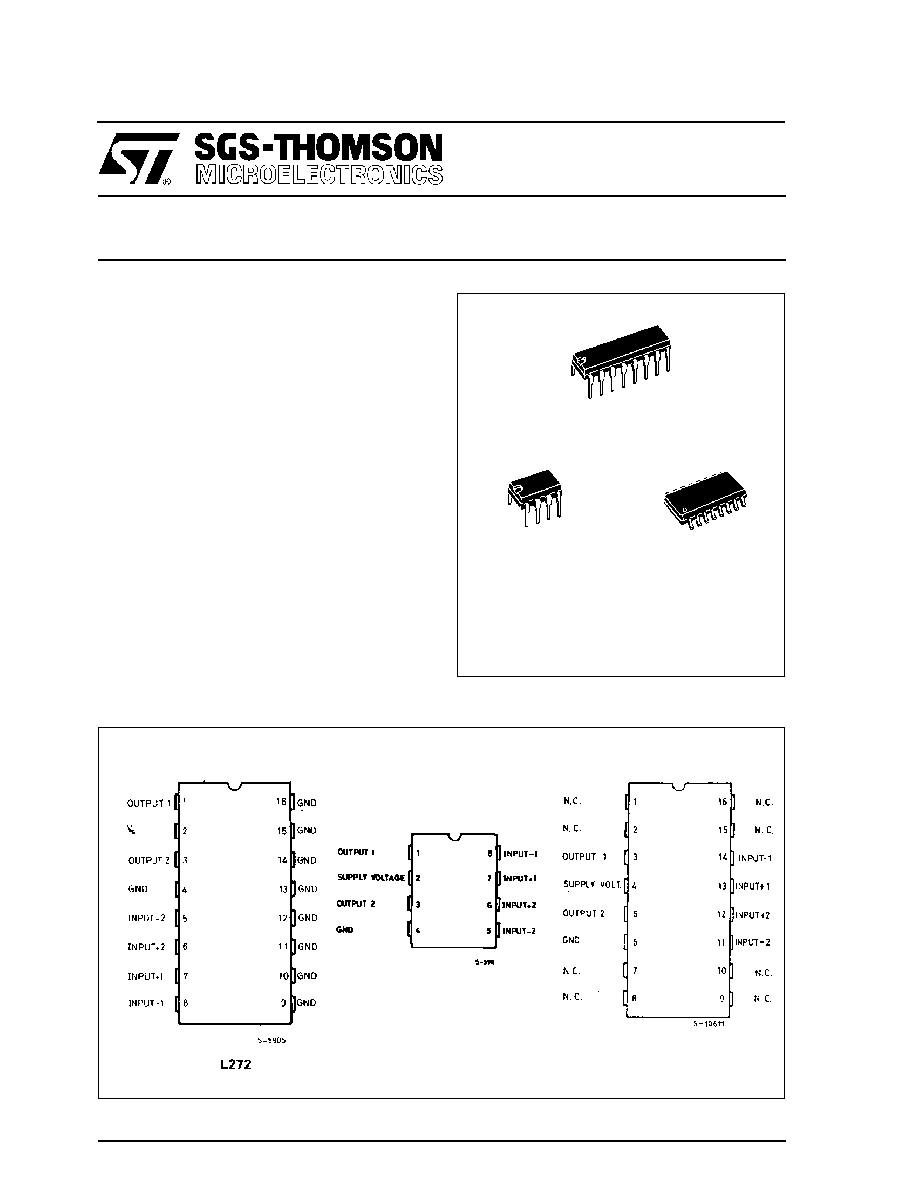L272
January 1995
DUAL POWER OPERATIONAL AMPLIFIERS
.
OUTPUT CURRENT TO 1 A
.
OPERATES AT LOW VOLTAGES
.
SINGLE OR SPLIT SUPPLY
.
LARGE COMMON-MODE AND DIFFEREN-
TIAL MODE RANGE
.
GROUND COMPATIBLE INPUTS
.
LOW SATURATION VOLTAGE
.
THERMAL SHUTDOWN
DESCRIPTION
The L272 is a monolithic integrated circuits in Pow-
erdip, Minidip and SO packages intended for use as
power operational amplifiers in a wide range of ap-
plications including servo amplifiers and power sup-
plies, compacts disc, VCR, etc.
The high gain and high output power capability pro-
vide superior performance whatever an operational
amplifier/power booster combination is required.
Powerdip
(8 + 8)
Minidip
ORDERING NUMBERS : L272 (Powerdip)
L272M (Minidip)
L272D (SO16 Narrow)
PIN CONNECTIONS (top view)
SO16 (Narrow)
L272D
L272M
1/10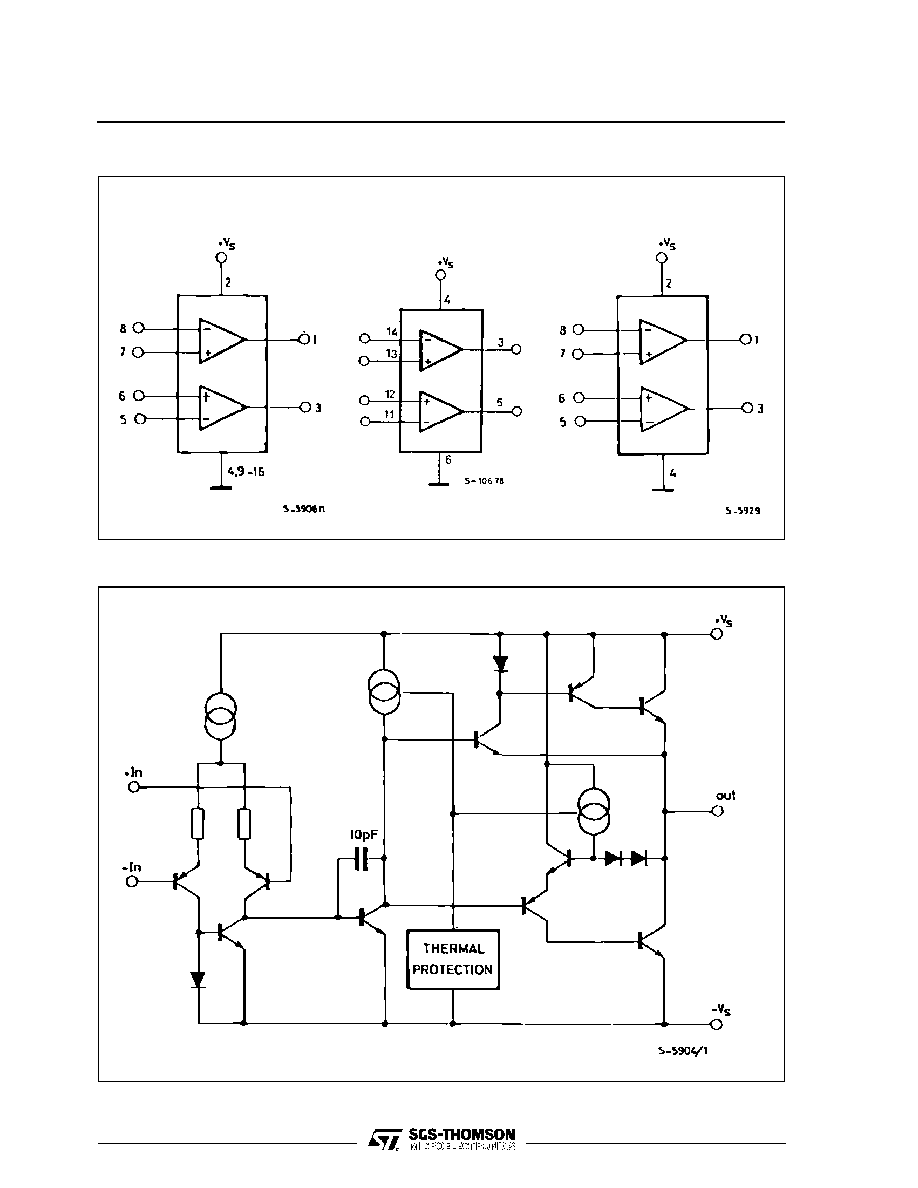BLOCK DIAGRAMS
SCHEMATIC DIAGRAM (one only)
L272
L272M
L272D
L272
2/10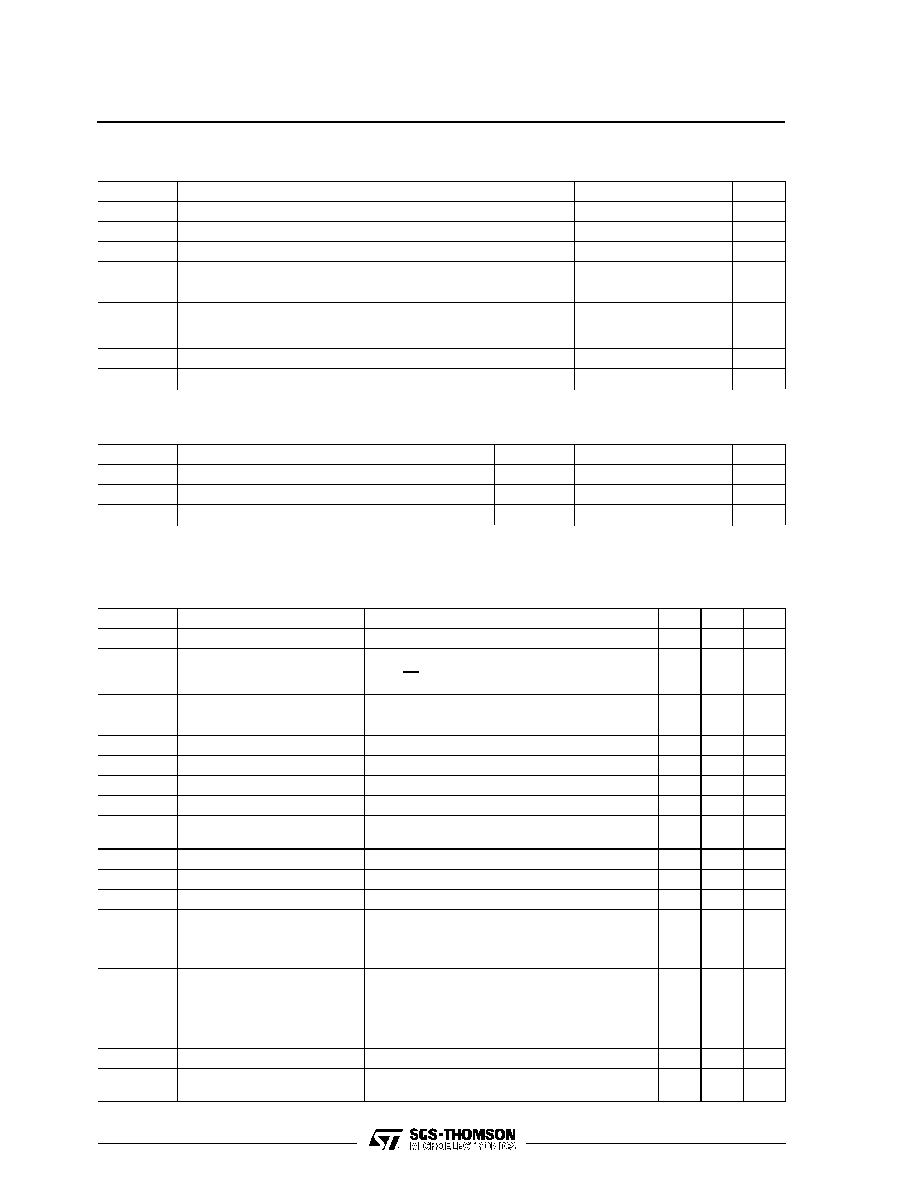ABSOLUTE MAXIMUM RATINGS
Symbol
Parameter
Value
Unit
V
s
Supply Voltage
28
V
V
i
Input Voltage
V
s
V
i
Differential Input Voltage
±
V
s
I
o
DC Output Current
1
A
I
p
Peak Output Current (non repetitive)
1.5
A
P
tot
Power Dissipation at:
T
amb
= 80
°
C (L272), T
amb
= 50
°
C (L272M), T
case
= 90
°
C (L272D)
T
case
= 75
°
C (L272)
1.2
5
W
W
T
op
Operating Temperature Range (L272D)
­ 40 to 85
°
C
T
stg
, T
j
Storage and Junction Temperature
­ 40 to 150
°
C
ELECTRICAL CHARACTERISTICS (V
S
= 24V, T
amb
= 25
o
C unless otherwise specified)
Symbol
Parameter
Test Conditions
Min.
Typ.
Max.
Unit
V
s
Supply Voltage
4
28
V
I
s
Quiescent Drain Current
V
O
=
V
S
2
V
s
= 24V
V
s
= 12V
8
7.5
12
11
mA
mA
I
b
Input Bias Current
0.3
2.5
µ
A
V
os
Input Offset Voltage
15
60
mV
I
os
Input Offset Current
50
250
nA
SR
Slew Rate
1
V/
µ
s
B
Gain-bandwidth Product
350
kHz
R
i
Input Resistance
500
k
G
v
O. L. Voltage Gain
f = 100Hz
f = 1kHz
60
70
50
dB
dB
e
N
Input Noise Voltage
B = 20kHz
10
µ
V
I
N
Input Noise Current
B = 20kHz
200
pA
CRR
Common Mode Rejection
f = 1kHz
60
75
dB
SVR
Supply Voltage Rejection
f = 100Hz, R
G
= 10k
, V
R
= 0.5V
V
s
= 24V
V
s
=
±
12V
V
s
=
±
6V
54
70
62
56
dB
V
o
Output Voltage Swing
I
p
= 0.1A
I
p
= 0.5A
21
23
22.5
V
V
C
s
Channel Separation
f = 1 kHz; R
L
=10
, G
v
= 30dB
V
s
= 24V
V
s
=
±
6V
60
60
dB
d
Distortion
f = 1kHz, G
v
= 3 dB, V
s
= 24V, R
L
=
0.5
%
T
sd
Thermal Shutdown Junction
Temperature
145
°
C
THERMAL DATA
Symbol
Parameter
Powerdip
SO16
Minidip
Unit
R
th j-case
Thermal Resistance Junction-pins
Max.
15
­
* 70
o
C/W
R
th j-amb
Thermal Resistance Junction-ambient
Max.
70
­
100
o
C/W
R
th j-alumina
Thermal Resistance Junction-alumina
Max.
­
** 50
­
o
C/W
*
Thermal resistance junction-pin 4
** Thermal resistance junctions-pins with the chip soldered on the middle of an alumina supporting substrate measuring
15x 20mm; 0.65mm thickness and infinite heatsink.
L272
3/10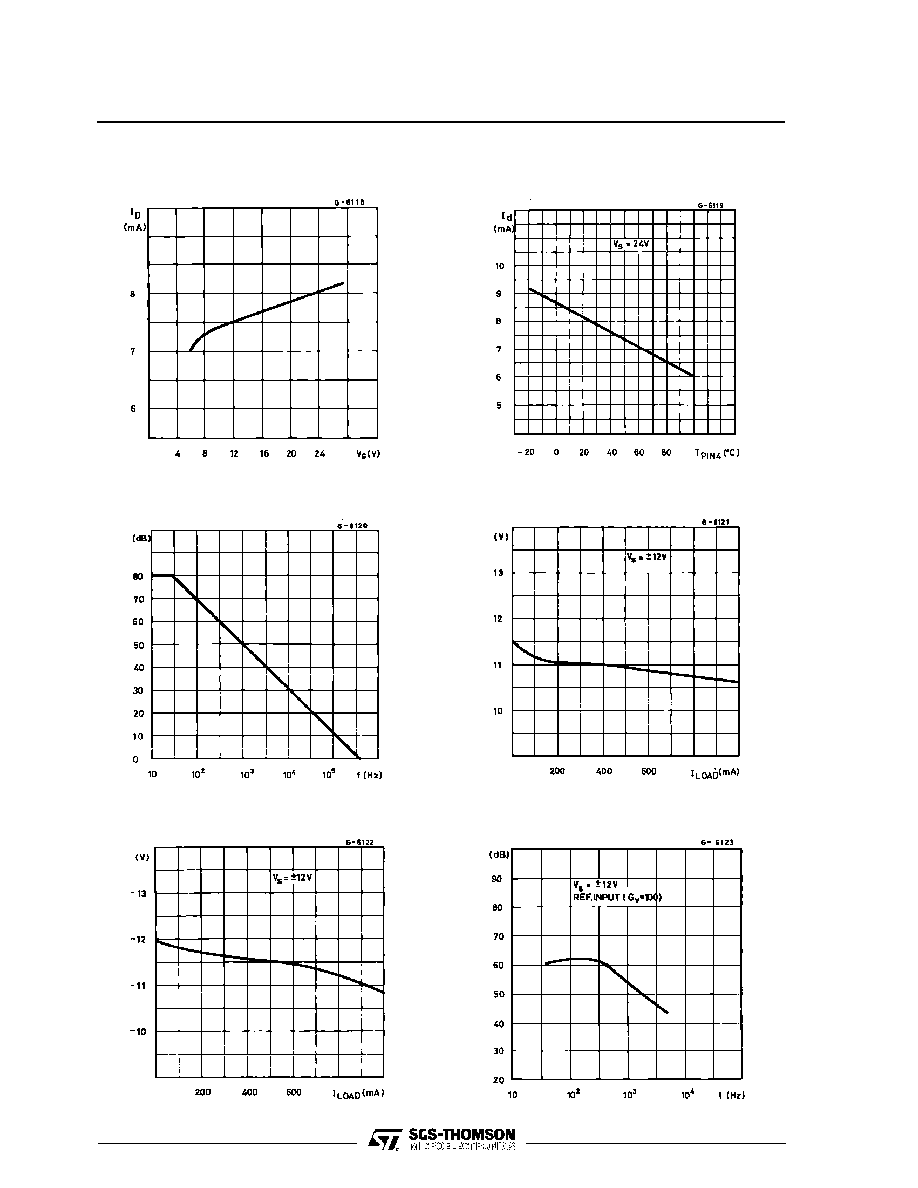Figure 1 :
Quiescent Current versus
Supply Voltage
Figure 2 :
Quiescent Drain Current versus
Temperature
Figure 3 :
Open Loop Voltage Gain
Figure 4 :
Output Voltage Swing versus
Load Current
Figure 5 :
Output Voltage Swing versus
Load Current
Figure 6 :
Supply Voltage Rejection versus
Frequency
L272
4/10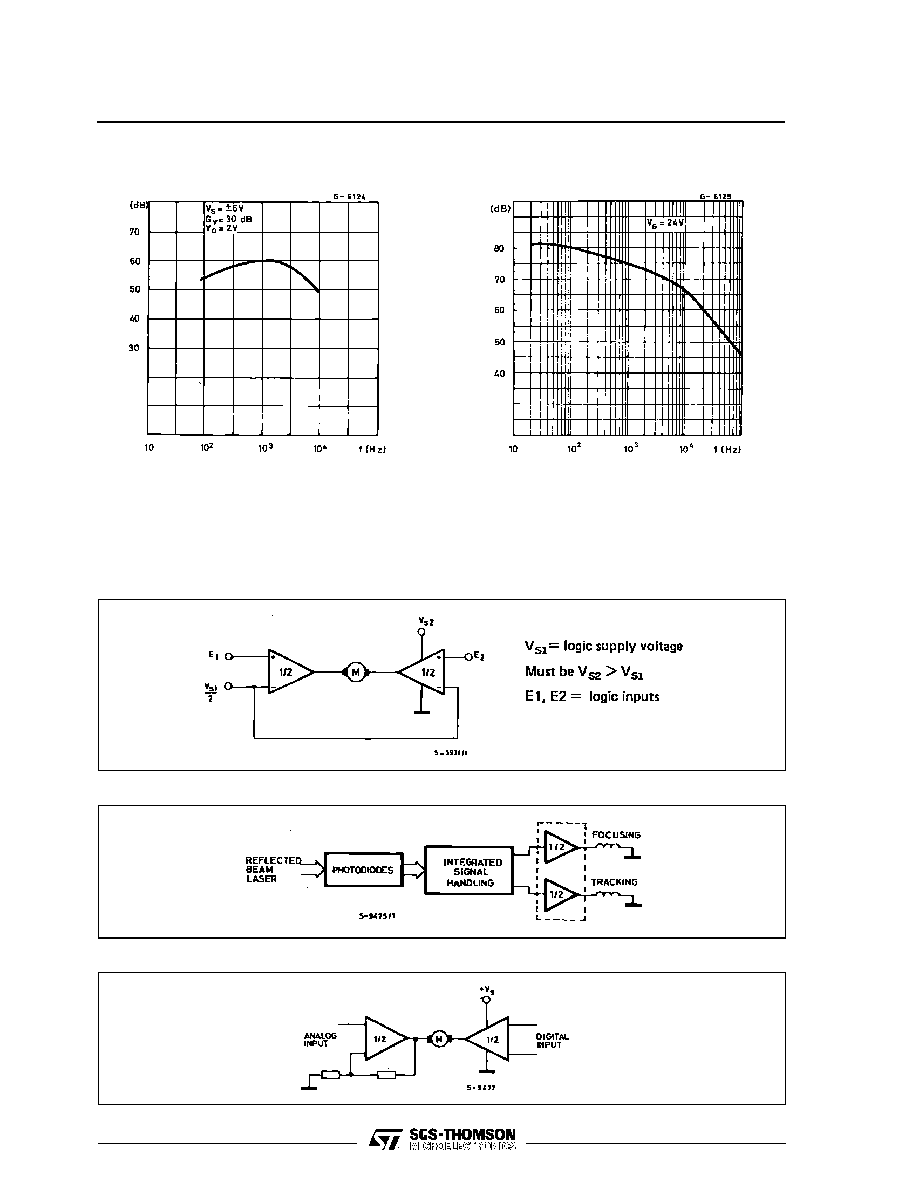Figure 7 :
Channel Separation versus
Frequency
Figure 8 :
Common Mode Rejection versus
Frequency
APPLICATION SUGGESTION
NOTE
In order to avoid possible instability occuring into fi-
nal stage the usual suggestions for the linear power
stages are useful, as for instance :
- layout accuracy ;
- a 100nF capacitor corrected between supply pins
and ground ;
- boucherot cell (0.1 to 0.2
µ
F + 1
series) between
outputs and ground or across the load.
Figure 9 : Bidirectional DC Motor Control with
µ
P Compatible Inputs
Figure 10 : Servocontrol for Compact-disc
Figure 11 : Capstan Motor Control in Video Recorders
L272
5/10© 2019 • ICSheet• Contact form• Main page